# Python JSON 模組 - 走到跑，跑到飛的 orjson

Posted on  May 10, 2023  in  Python 模組/套件推薦  by  Amo Chen  ‐ 4 min read

orjson 是一套由 Rust 實作的 Python 套件，專門用以處理 JSON 相關的 encode 與 decode 的工作，效率不僅快（根據官方測試最快可以達到 json 模組的 40 到 50 倍效率）更天生能直接處理 Python 內建 json 模組所無法序列化(serialize)的 datetime, UUID, dataclass 等資料，不需額外編寫序列化的處理程式。

### 前言 #

Python 雖然有內建 json 模組供開發者處理 JSON 格式的資料，例如將資料輸出為 JSON 格式，或將 JSON 字串載入並轉為 Python 資料。

### 本文環境 #

``````＄ pip install orjson
``````

### 內建 json 模組 vs. orjson #

``````import json

from datetime import datetime

d = {
'time': datetime.now(),
}

j = json.dumps(d)
``````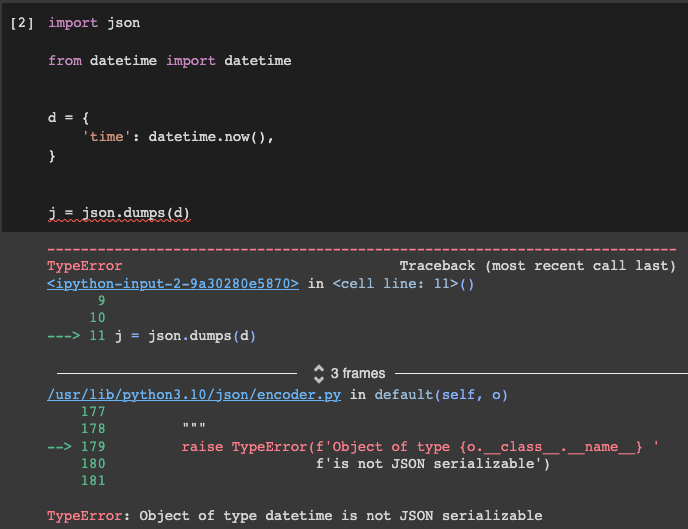``````import orjson

from datetime import datetime

d = {
'time': datetime.now(),
}

j = orjson.dumps(d)
print(j)
``````

``````b'{"time":"2023-05-10T02:36:43.813289"}'
``````

``````orjson.dumps({'time': datetime.now()}).decode()
``````

``````with open('output.jsonlines', 'wb') as file:
file.write(orjson.dumps(d, option=orjson.OPT_APPEND_NEWLINE)
``````

``````import orjson
from datetime import datetime

j = orjson.dumps(datetime.now(), option=orjson.OPT_OMIT_MICROSECONDS)
print(j)
``````

``````b'"2023-05-10T05:51:31"'
``````

orjson 也能正常處理含有時區(tzinfo)的 datetime 資料, 例如：

``````import orjson, datetime, zoneinfo

orjson.dumps(
datetime.datetime(
2023, 5, 1, 2, 3, 4, 5,
tzinfo=zoneinfo.ZoneInfo('Asia/Taipei'),
)
)
``````

``````b'"2022-12-01T02:03:04.000009+08:00"'
``````

orjson 內建多種原本 Python json 模組無法處理的資料，例如：

``````target = {
"message": "Hello, JSON",
"count": 10,
}
``````

``````%time json.dumps(target)
%time orjson.dumps(target)
``````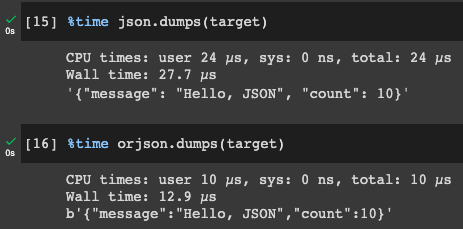#### dataclass #

``````import json

from dataclasses import dataclass

@dataclass
class User(object):
uid: int
name: str
role: str

json.dumps(user)
``````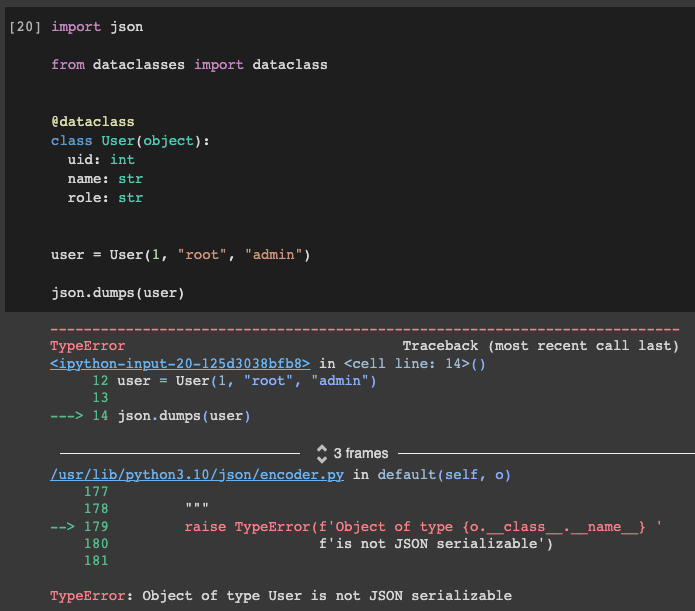``````import orjson

from dataclasses import dataclass

@dataclass
class User(object):
uid: int
name: str
role: str

orjson.dumps(user)
``````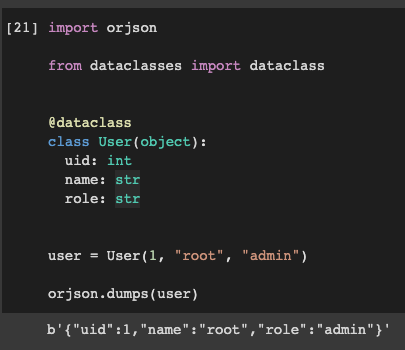### numpy #

numpy 的 JSON 序列化也是 orjson 所主打的功能特點，不過 orjson 預設並沒有開啟 numpy 序列化的功能，如下列範例會出現 `TypeError`

``````import orjson, numpy

orjson.dumps(
numpy.array([[1, 2, 3], [4, 5, 6]]),
)
``````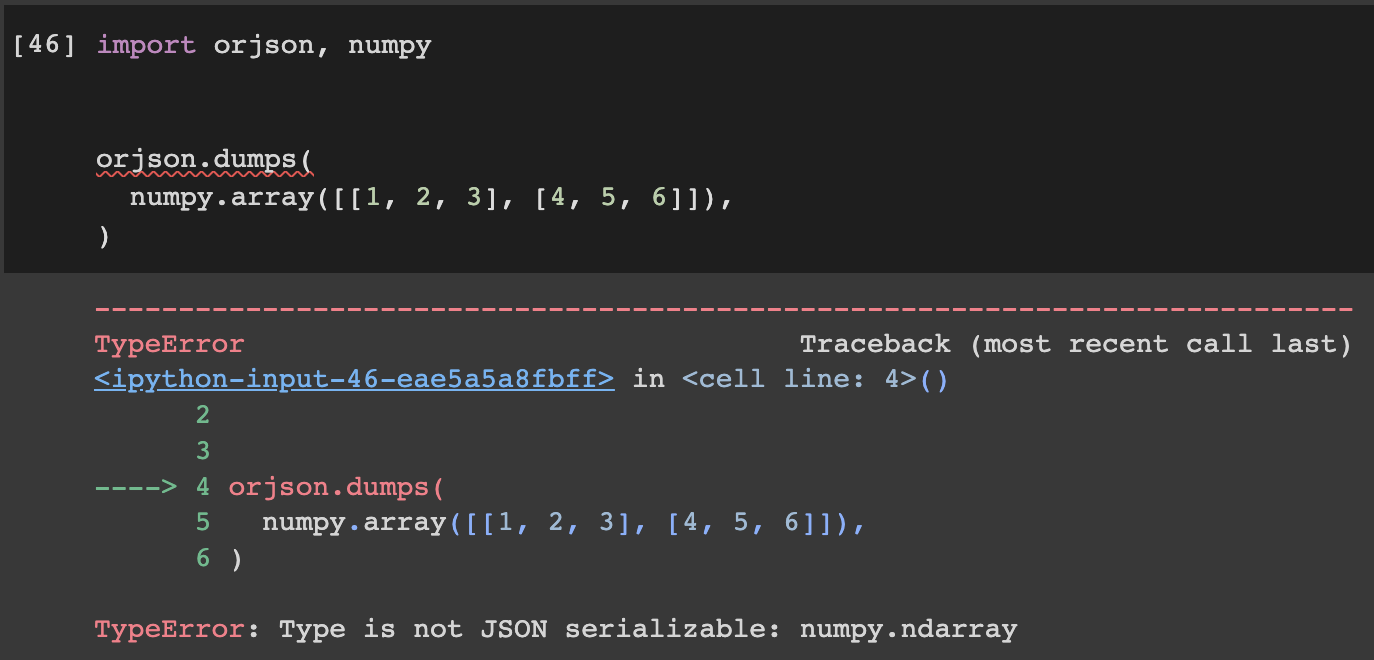``````import orjson, numpy

orjson.dumps(
numpy.array([[1, 2, 3], [4, 5, 6]]),
option=orjson.OPT_SERIALIZE_NUMPY,
)
``````

### orjson.loads() 載入 JSON 資料 #

``````orjson.loads('{"uid":1,"name":"root","role":"admin"}')
``````

``````orjson.loads(b'{"uid":1,"name":"root","role":"admin"}')
``````

``````with open('input.jsonlines', 'rb') as file:
for line in file:
``````

### default 參數 #

``````import orjson, decimal

orjson.dumps(decimal.Decimal("0.123"))
````````````import orjson, decimal

def default(obj):
if isinstance(obj, decimal.Decimal):
return str(obj)
raise TypeError

orjson.dumps(decimal.Decimal("0.123"), default=default)
``````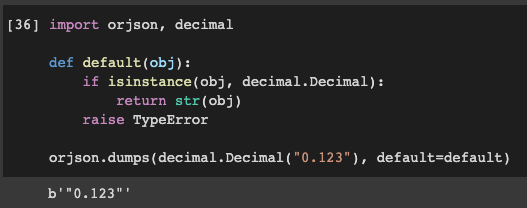### 總結 #

orjson 憑藉著 Rust 實作的效能優勢以及支援各種常見的資料型態序列化，不僅能夠減少 Python 開發者的負擔，也能有效提升 JSON 相關應用執行速度。

``````import orjson as json
``````

GitHub - ijl/orjson: Fast, correct Python JSON library supporting dataclasses, datetimes, and numpy